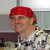## Lotus Formula @ifTags:
Lotus
Lotus development
Lotus Notes 6.5.x
Have a subform that I am trying to set the value of Tax based on a plant field and useage. If the Plant is 3 then tax is 5% for everthing. If Plant is 1 or 2 then tax is 7% on non production and 0% on production.

Here is the code for the Tax field.

@If(Type3 != "Plant#3") & @If(Prod != "Production");@Sum(SubTotal * 0.07);@If(Type3 = "Plant#3");@Sum(SubTotal * 0.05);0

Software/Hardware used:
lotus notes 6.5.4

Thanks. We'll let you know when a new response is added.

Try this:

@If(Type3 != “Plant#3”; SubTotal * 0.05; @If(Prod != “Production”; SubTotal * 0.07; 0))

## Discuss This Question: 2 Replies

•